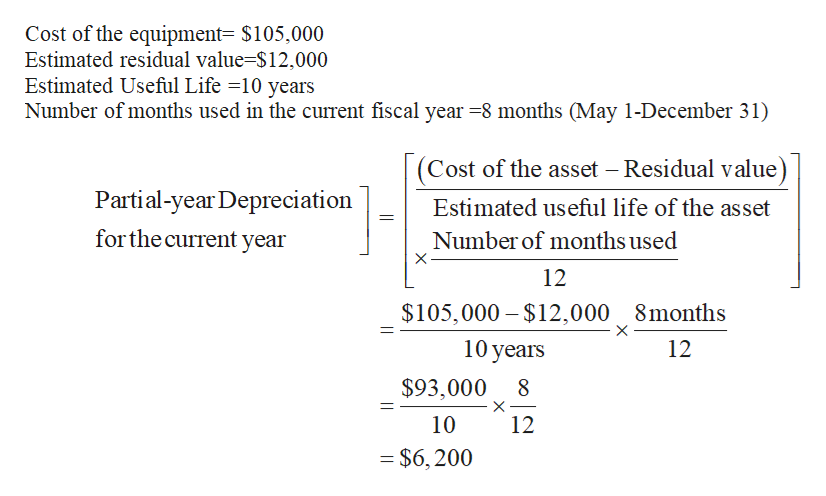# Equipment acquired at a cost of \$105,000 has an estimated residual value of \$12,000 and an estimated useful life of 10 years. It was placed into service on May 1 of the current fiscal year, which ends on December 31. Determine the depreciation for the current fiscal year and for the following fiscal year by (a) the straight-line method and (b) the double-declining-balance method.

Question
27 views

Equipment acquired at a cost of \$105,000 has an estimated residual value of \$12,000 and an estimated useful life of 10 years. It was placed into service on May 1 of the current fiscal year, which ends on December 31. Determine the depreciation for the current fiscal year and for the following fiscal year by (a) the straight-line method and (b) the double-declining-balance method.

check_circle

Step 1

Straight-line Depreciation:

Under the straight-line method of depreciation, the same amount of depreciation is allocated every year over the estimated useful life of an asset.

Double-declining-balance method:

It is an accelerated method of depreciation under which the depreciation declines in each successive year until the value of asset becomes zero. Under this method, the book value (original cost less accumulated depreciation) of the long-term asset is decreased by a fixed rate. It is double the rate of the straight-line depreciation.

Step 2

Requirement a:

Compute the amount of depreciation by the straight-line method for the current fiscal year and for the following fiscal year.

Determine the amount of depreciation for the current fiscal year.help_outlineImage TranscriptioncloseCost of the equipment= \$105,000 Estimated residual value=\$12,000 Estimated Useful Life =10 years Number of months used in the current fiscal year =8 months (May 1-December 31) (Cost of the asset – Residual value) Partial-year Depreciation Estimated useful life of the asset for the current year Number of months used 12 \$105,000 – \$12,000 8months 10 years 12 \$93,000 10 12 = \$6,200 fullscreen
Step 3

Determine the amount of depreciation for the ...

### Want to see the full answer?

See Solution

#### Want to see this answer and more?

Solutions are written by subject experts who are available 24/7. Questions are typically answered within 1 hour.*

See Solution
*Response times may vary by subject and question.
Tagged in